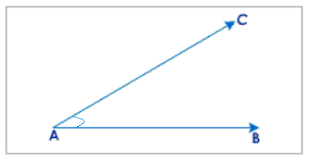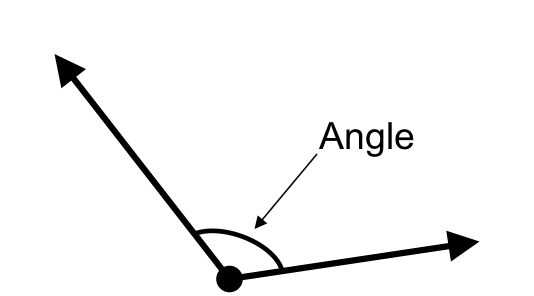Angles Types (4.MD.6)
starstarstarstarstarstarstarstarstarstar
by Tina Walker Simmons
| 7 Questions1
1 pt
What type of angle is this?
Acute
Obtuse
Right
Triangle2
1 pt
It's obtuse.
It's measurement is greater than 90 degrees.
It's measurement is less than 90 degrees.
It is an acute angle.3
1 pt
What is true about the angle above? (Mark all that are true.)
This is a right angle.
This is a straight angle.
An obtuse angle is greater than this angle.
An acute angle is greater than this angle.
4
1 pt
Draw an angle that could be 100 degrees. (Use the line tool.)
5
1 pt
Draw an angle that could be 45 degrees. (Use the line tool.)
6
1 pt
Draw an angle that could be 90 degrees. (Use the line tool.)
7
1 pt
These are all examples of .... (Choose all that are true.)
Obtuse angles.
Right angles.
Acute angles
Lines